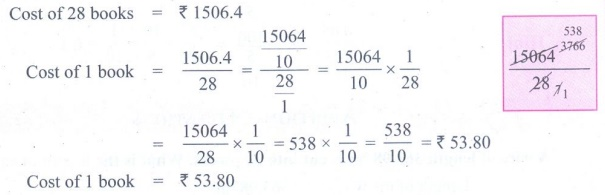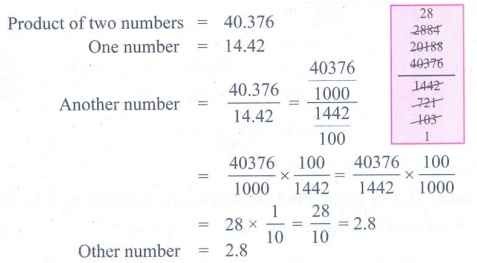Home | | Maths 7th Std | Exercise 1.4 (Division of Decimal Numbers)

# Exercise 1.4 (Division of Decimal Numbers)

7th Maths : Term 3 Unit 1 : Number System : Division of Decimal Numbers : Exercise 1.4

Operations on Decimal Numbers

Division of Decimal Numbers

Exercise 1.4

1. Simplify the following.

(i) 0.6 ÷ 3

(ii) 0.90 ÷ 5

(iii) 4.08 ÷ 4

(iv) 21.56 ÷ 7

(v) 0.564 ÷ 6

(vi) 41.36 ÷ 4

(vii) 298.2 ÷ 3

Solution:2. Simplify the following.

(i) 5.7 ÷ 10

(ii) 93.7 ÷ 10

(iii) 0.9 ÷ 10

(iv) 301.301 ÷ 10

(v) 0.83 ÷ 10

(vi) 0.062 ÷ 10

Solution:3. Simplify the following.

(i) 0.7 ÷ 100

(ii) 3.8 ÷ 100

(iii) 49.3 ÷ 100

(iv) 463.85 ÷ 100

(v) 0.3 ÷ 100

(vi) 27.4 ÷ 100

Solution:4. Simplify the following.

(i) 18.9 ÷ 1000

(ii) 0.87 ÷ 1000

(iii) 49.3 ÷ 1000

(iv) 0.3 ÷ 1000

(v) 382.4 ÷ 1000

(vi) 93.8 ÷ 1000

Solution:5. Simplify the following.

(i) 19.2 ÷ 2.4

(ii) 4.95 ÷ 0.5

(iii) 19.11÷ 1.3

(iv) 0.399 ÷ 2.1

(v) 5.4 ÷ 0.6

(vi) 2.197 ÷ 1.3

Solution:6. Divide 9.55 kg of sweet among 5 children. How much will each child get?

Solution:7. A vehicle covers a distance of 76.8 km for 1.2 litre of petrol. How much distance will it cover for one litre of petrol?

Solution:8. Cost of levelling a land at the rate of ₹ 15.50 sq. ft is ₹ 10,075. Find the area of the land.

Solution:9. The cost of 28 books are ₹ 1506.4. Find the cost of one book.

Solution:10. The product of two numbers is 40.376. One number is 14.42. Find the other number.

Solution:Objective type questions

11. 5.6÷ 0.5= ?

(i) 11.4

(ii) 10.4

(iii) 0.14

(iv) 11.2

Hint:

5.6 / 0.5 = 56 / 5

= 11.212. 2.01÷ 0.03 = ?

(i) 6.7

(ii) 67.0

(iii) 0.67

(iv) 0.067

Hint:

2.01 / 0.03 = 201 / 3

= 6713. 0.05 ÷ 0.5 = ?

(i) 0.01

(ii) 0.1

(iii) 0.10

(iv) 1.0

Solution: 0.05 / 0.5 = (5/100) / (5 / 10) = 5 /100 × 10/5 = 1 /10 = 0.1

Exercise 1.4

1. (i) 0.2 (ii) 0.18 (iii) 1.02 (iv) 3.08 (v) 0.094 (vi) 10.34 (vii) 99.4

2. (i) 0.57 (ii) 9.37 (iii) 0.09 (iv) 30.1301  (v) 0.083 (vi) 0.0062

3. (i) 0.007 (ii) 0.038 (iii) 0.493 (iv) 4.6385 (v) 0.003 (vi) 0.274

4. (i) 0.0189 (ii) 0.00087 (iii) 0.0493 (iv) 0.0003 (v) 0.3824 (vi) 0.0938

5. (i) 8 (ii) 9.9 (iii) 14.7 (iv) 0.19 (v) 9 (vi) 1.69

6. 1.91 kg

7. 64 km

8. 650 sq.ft

9. ₹ 53.80

10. 2.8

Objective type questions

11. (iv) 11.2

12. (ii) 67.0

13. (ii) 0.1

Operations on Decimal Numbers

Division of Decimal Numbers

Exercise 1.4

1. Simplify the following.

(i) 0.6 ÷ 3

(ii) 0.90 ÷ 5

(iii) 4.08 ÷ 4

(iv) 21.56 ÷ 7

(v) 0.564 ÷ 6

(vi) 41.36 ÷ 4

(vii) 298.2 ÷ 3

Solution:i) 0.6 ÷ 3 = 6/10 × 1/3 = 6/3 × 1/10 = 2 × 1/10 = 2/10 = 0.2

(ii) 0.90 ÷ 5 = 90/100  ×  1/5 = 90/5 × 1/100 = 18 × ( 1/100) = 18/100 = 0.18

(iii) 4.08 ÷ 4 = 408/100 × 1/4 = 408/4   ×   1/100 = 102 × ( 1/100) = 102/100 = 1.02

(iv) 21.56 ÷ 7 =   2156/100 × 1/7 = 2156/7  ×  1/100 = 102 × (1/100) = 308/100 = 3.08

(v) 0.564 ÷ 6 = 564/1000 × 1/6 = ( 564/6) × ( 1/1000) = 94/1000 = 0.094.

(vi) 41.36 ÷ 4 = 4136 /100 × 1/4 = (4136/4) × 1/100 = 1034/100 = 10.34

(vii) 298.2 ÷ 3 = 2982/10 × 1/3 =( 2982 /3) × 1/10 = 994/10 = 99.4

2. Simplify the following.

(i) 5.7 ÷ 10

(ii) 93.7 ÷ 10

(iii) 0.9 ÷ 10

(iv) 301.301 ÷ 10

(v) 0.83 ÷ 10

(vi) 0.062 ÷ 10

Solution:(i) 5.7 ÷ 10 = 57/10 × 1/10 = 57/100 = 0.57

(ii) 93.7 ÷ 10 = 937/10 × 1/10 = 937/100 = 9.37

(iii) 0.9 ÷ 10 = 9/10 × 1/10 = 9/100 = 0.09

(iv) 301.301 ÷ 10 = (301301/1000 )x 1/10 = 301301/10000 = 30.1301

(v) 0.83 ÷ 10 = (83/100 )x 1/10 = 83/1000 = 0.083

(vi) 0.062 ÷10 =( 62/1000) × 1/10 = 62/10000 = 0.0062

3. Simplify the following.

(i) 0.7 ÷ 100

(ii) 3.8 ÷ 100

(iii) 49.3 ÷ 100

(iv) 463.85 ÷ 100

(v) 0.3 ÷ 100

(vi) 27.4 ÷ 100

Solution:(i) (i) 0.7 ÷ 100 = 7/10 × 1/100 = 7/1000 = 0.007

(ii) 3.8 ÷ 100 = 38/10 × 1/100 = 38/1000 = 0.038

(iii) 49.3 ÷ 100 = 493/10 × 1/100 = 493/1000 = 0.493

(iv) 463.85 ÷ 100 = (46385/100) × 1/100 = 46385/10000 = 4.6385

(v) 0.3 ÷ 100 = 3/10 × 1/100 = 3/ 1000 = 0.003

(vi) 27.4 ÷ 100 = 274/10 × 1/100 = 274/1000 = 0.274

4. Simplify the following.

(i) 18.9 ÷ 1000

(ii) 0.87 ÷ 1000

(iii) 49.3 ÷ 1000

(iv) 0.3 ÷ 1000

(v) 382.4 ÷ 1000

(vi) 93.8 ÷ 1000

Solution:(i) 18.9 ÷ 1000 = 189/10 × 1/1000 = 189/10000 = 0.0189

(ii) 0.87÷ 1000 = 87/100 × 1/1000 = 87/ 100000 = 0.00087

(iii) 49.3 ÷1000 = 493/10 × 1/100 = 493/ 1000 = 0.493

(iv) 0.3÷ 1000 = 3/10 × 1/1000 = 3/10000 = 0.0003

(v) 382.4 ÷1000 = 3824/10 × 1/1000 = 3824/10000 = 0.3824

(vi) 93.8 ÷1000 = 938/10 × 1/1000 = 938/10000 = 0.0938

5. Simplify the following.

(i) 19.2 ÷ 2.4

(ii) 4.95 ÷ 0.5

(iii) 19.11÷ 1.3

(iv) 0.399 ÷ 2.1

(v) 5.4 ÷ 0.6

(vi) 2.197 ÷ 1.3

Solution:(i) 19.2 ÷ 2.4 = ( 192/10) / ( 24/10 ) = 192/10 × 10/24 = 192/24 = 8

(ii) 4.95 ÷ 0.5 = ( 495/100) / ( 5/10 ) = 495/100 × 10/5 = 495/5 × 10/100 = 99 × 1/10 = 99/10 = 9.9

(iii) 19.11 ÷ 1.3 = ( [19 × 11]/100)/( 13/10 ) = [1911/100] × [10/13]

= 1911/13 × 10/100 = 147 × 1/10 = 147/10 = 14.7

(iv) 0.399 ÷ 2.1 = (399/1000) / (21/10) = 399/1000 × 10/21

= 399/21 × 10/1000

= 19 × 1/100 = 19/100 = 0.19

(v) 5.4 ÷ 0.6

= (54/10) / (6/10) = 54/10 × 10/6

= 54 / 6 = 9

(vi) 2.197 ÷ 1.3

= (2197/1000) / (13/10) = 2197/1000 × 10/13

= 169 × 1/100

= 169/100 = 1.69

6. Divide 9.55 kg of sweet among 5 children. How much will each child get?

Solution:Weight of the sweet = 9.55 kg

Weight of sweet for 5 children = 955/100 kg

Weight of sweet for 1 child = (955/100) / 5 = ( 955/100) × 1/5 =( 955 / 5) × 1/100

= 191/100 = 1.91

Each child will get 1.91 kg sweet.

7. A vehicle covers a distance of 76.8 km for 1.2 litre of petrol. How much distance will it cover for one litre of petrol?

Solution:For 1.2 litre of petrol the distance covered = 76.8 km  = 768 / 10 km

For 1 litre of petrol distance covered = [ (768 / 10) / 1.2 ] km

= (768 / 10) / (12 / 10) = ( 768 / 10) × (10 / 12)

= (768 / 12) × (10 / 10) = 64 km

For 1 litre of petrol distance covered = 64 km.

8. Cost of levelling a land at the rate of ₹ 15.50 sq. ft is ₹ 10,075. Find the area of the land.

Solution:Cost of levelling the entire land = ₹ 10, 075

Cost of levelling 1 sq. ft = ₹ 15.50

Area of the land = Cost of levelling the entire land / Cost of levelling 1 sq. ft.

=> (10075 / 15.50) × (100 / 100) = ( 10075 × 100) / (15.50 × 100) = 1007500 / 1550

= 100750 / 155 = 650

Area of the land = 650 sq.ft.

9. The cost of 28 books are ₹ 1506.4. Find the cost of one book.

Solution:Cost of 28 books = ₹ 1506.4

Cost of 1 book = 1506.4 / 28 = (15064 / 10) / ( 28 / 1) = (15064 / 10) × (1 / 28)

= (1506.4 / 28) × (1 / 10) = 538 × ( 1 / 10 ) = 538 / 10 = ₹ 53.80

Cost of 1 book = ₹ 53.80

10. The product of two numbers is 40.376. One number is 14.42. Find the other number.

Solution:Product of two numbers = 40.376

One number = 14.42

Another number = 40376 / 14.42 = (40376 / 1000) / ( 1442 / 100)

= (40376 / 1000) × (100 / 1442) = ( 40376 / 1442) × (100 / 1000)

= 28 × (1 /10) = 28 / 10 = 2.8

Other number = 2.8

Objective type questions

11. 5.6÷ 0.5= ?

(i) 11.4

(ii) 10.4

(iii) 0.14

(iv) 11.2

Hint:

5.6 / 0.5 = 56 / 5

= 11.212. 2.01÷ 0.03 = ?

(i) 6.7

(ii) 67.0

(iii) 0.67

(iv) 0.067

Hint:

2.01 / 0.03 = 201 / 3

= 6713. 0.05 ÷ 0.5 = ?

(i) 0.01

(ii) 0.1

(iii) 0.10

(iv) 1.0

Solution: 0.05 / 0.5 = (5/100) / (5 / 10) = 5 /100 × 10/5 = 1 /10 = 0.1

Exercise 1.4

1. (i) 0.2 (ii) 0.18 (iii) 1.02 (iv) 3.08 (v) 0.094 (vi) 10.34 (vii) 99.4

2. (i) 0.57 (ii) 9.37 (iii) 0.09 (iv) 30.1301  (v) 0.083 (vi) 0.0062

3. (i) 0.007 (ii) 0.038 (iii) 0.493 (iv) 4.6385 (v) 0.003 (vi) 0.274

4. (i) 0.0189 (ii) 0.00087 (iii) 0.0493 (iv) 0.0003 (v) 0.3824 (vi) 0.0938

5. (i) 8 (ii) 9.9 (iii) 14.7 (iv) 0.19 (v) 9 (vi) 1.69

6. 1.91 kg

7. 64 km

8. 650 sq.ft

9. ₹ 53.80

10. 2.8

Objective type questions

11. (iv) 11.2

12. (ii) 67.0

13. (ii) 0.1

Tags : Questions with Answers, Solution | Number System | Term 3 Chapter 1 | 7th Maths , 7th Maths : Term 3 Unit 1 : Number System
Study Material, Lecturing Notes, Assignment, Reference, Wiki description explanation, brief detail
7th Maths : Term 3 Unit 1 : Number System : Exercise 1.4 (Division of Decimal Numbers) | Questions with Answers, Solution | Number System | Term 3 Chapter 1 | 7th Maths